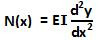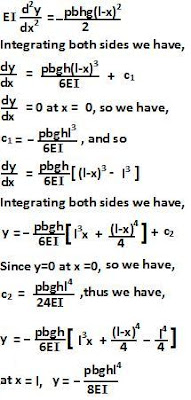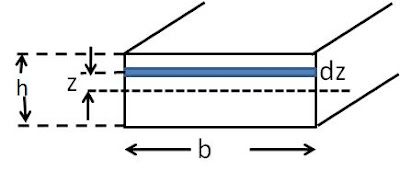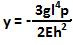## Saturday, April 11, 2009

### Irodov Problem 1.303

a)Let the width of the cantilever be b, its height h, length l and density p.
Consider the torques acting on a section of the cantilever OB as shown in the figure. There are two moments acting on this section of the cantilever, i) due to its own weight phb(l-x)g acting at the center of the section at a distance (l-x)/2 from O in a clockwise direction and ii) the elastic moment N(x) generated by the curvature of the cantilever. From Irodov problem 1.301 (derivation at this link) we know that,Since the cantilever is at equilibrium, these moments must cancel each other. Thus, N(x) must act in the anti-clockwise direction. This in turn means that the upper surface of the cantilever OB must be stretched more than the lower surface O'B' as shown in the figure. In other words, the elastic curve of the cantilever must be a concave function i.e..

Hence, we have,Now the moment of inertia I about the neutral axis of the
cantilever can be obtained as,Using (1) we can obtain the depression y as,. The negative sign indicating depression.

b)If the width of the beam is b as in part a), the total weight of the beam is 2bphlg. In order for the beam to be in equilibrium, the net reaction force from the end points must be equal to 2bphlg acting upwards. Further, the reaction from each of the end points should be exactly same since otherwise a net torque will be induced in the beam about the center of the beam. Thus, each end point provides a reaction of bplgh as shown in the figure.

Now let us consider a small section of the beam AO that is x units long, as shown in the figure. The net weight of this section of the beam is bpghx and acts at the center of this section at a distance of x/2 from the end point A.

There are three torques acting on the beam about point O, i) due to reaction from the end point A given by lpbghx in the clockwise direction, ii) due the weight of the beam given byand acts in the anti-clockwise direction and finally iii) the elastic moment N(x) due to the curvature in the beam.

N(x) must completely balance the sum of the other two torques and thus must act in the anti-clockwise direction (since the torque acting at the end point is greater than the torque due to the weight). This can only happen if the bottom surface of the beam AO is stretched more than the top surface of the beam A'O' as seen in the figure. In other words, the elastic curve of the beam will be a convex function i.e..

Hence we have,